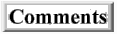Quantum Mechanics is a Perfect Process

Home

TOC

In retrospect, it seems quantum mechanics could have been derived just out of considerations of quality control. And there were even hints of this by the ancient Greeks with their theory that matter is composed of atoms. For what did they mean by atoms? They meant that atoms were the smallest constituents of a material substance that had the properties of that material. Every atom of that material would be measured to have exactly the same properties no matter how many times you measured it. If the properties of the atom changed at all, then you'd be talking about a different substance. The material in a sense is quantized into atom, the first quantum theory.

This in itself is enough information to anticipate the modern theory of quantum mechanics. For imagine that you are making something to sell on the market and you want to measure the tolerances of your parts to see how exact your manufacturing process is. What you'd do is measure each part and put them in bins separated into subintervals of the possible values your measurement could take. Perhaps you did this exercise in high-school. Then you would count the relative number of parts that were in each bin and draw a graph of the probability distribution for that process. Normally, you'd get a bell curve, or gaussian distribution, if only random noise was responsible for the differences in measurement. The width of this bell curve is measured in terms of a number called the standard deviation. The smaller the standard deviation, the more exact your manufacturing process and the more uniform your parts are made.

Now imagine that you were able to fix your process to reduce the effects of any noise. Your tolerance would be getting tighter and tighter. Your distribution graph would begin to look like a larger and larger spike at a single value that drops off quickly on either side of the center. And in a perfect process, all the parts would be exactly the same. So if you were to measure an infinite number of parts, you'd get all of them at a single value. It would begin to look like a Dirac delta function.

This is exactly what atoms are. If you measured the relevant property of atoms of the same substance, they would all have exactly the same value (of mass or charge, etc.). They would be represented by a Dirac delta function. So we should expect to use the math associated with the Dirac delta to describe this kind of quantum theory. And when we considered how one atom related to another atom, then we would have to consider the math of how one Dirac delta function was related to other Dirac delta functions. This would almost immediately lead to the Path Integral of quantum mechanics that describes a wave function as shown next.

The Dirac delta is defined as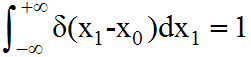And for any function f(x), we get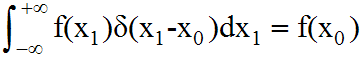See dirac delta function at Wikipedia for eq  and eq  above.

But if f(x1) is the Dirac delta function itself, then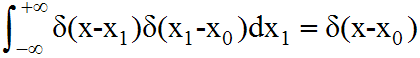See reference 1) for eq .

Equation  is a recursive relation that can be applied as many time as desired. Applying it again gives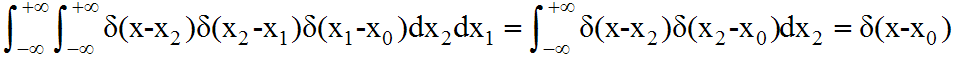And applying it an infinite number of times gives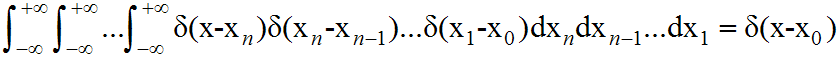See reference 2) for eq .

And if we are talking about near perfect manufacturing process that have gaussian distributions, then we should let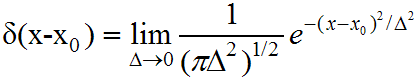with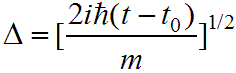{See reference 3) for eq }

It remains to be seen why the gaussian in  should be complex, but an argument can be made based on algebraic properties of multiplying distributions as shown here. It also remains to be seen why m and h-bar are introduced, but they can be seen as constants setting units of measure. They could have been set to 1 without loss of generality. And allowing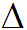to be express in terms of (t-t0), with t and t0 related to x and x0, allows us to relate one Dirac delta function to another in eq.

Then rearranging eq, we get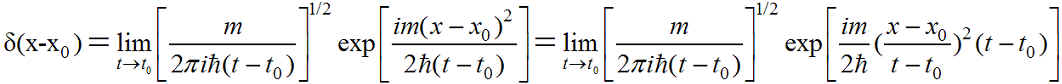which equals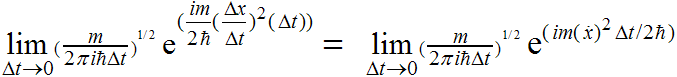We see then that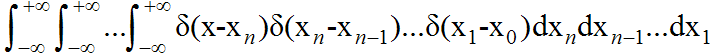is equal to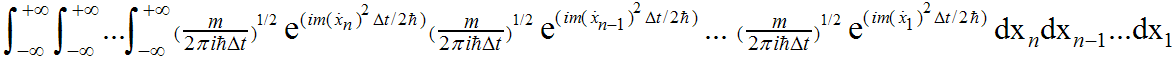And by gathering terms in the exponential, this is equal to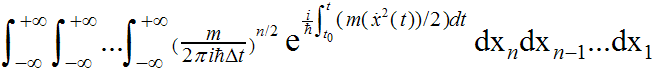which is the Feynman Path Integral formulation of quantum mechanics for a single particle with no potential in one dimension. See reference 3), page 238, eq 8.4.3 and reference 4), page 42, eq 3.2 and reference 2), page 100, eq 2.64 with V=0.

This is usually shortened to the notation: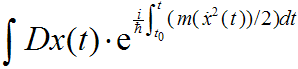Equation  gives us the wavefunction of quantum mechanics. And as shown above this could have been anticipated early on. I suspect that theoretical physicists back then were not accustomed to thinking about quality control issues in manufacturing process. Or perhaps they thought that no manufacturing process could ever approach perfection, not even by nature. However, in retrospect, this is now easy to see. It seems nature manufactures electrons with exactly the same measured properties, and protons with exactly the same properties.

Home

References:

1) Quantum Field Theory, by Lowell S. Brown, page 30, eq 1.6.4.

2) Path Integrals in Quantum Mechanics, Statistics, Polymer Physics, and Financial Markets, 4th Edition, by Hagen Kleinert, page 91, eq 2.17.

3) Principles of Quantum Mechanics, by Shankar and Plenum, page 236.

4) Quantum Mechanics and Path Integrals, by Richard P. Feynman and Albert R. Hibbs, Emended by Daniel F. Styer, page 43.